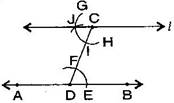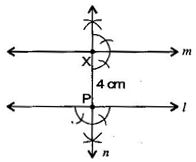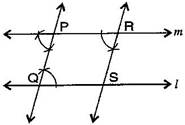### Practical Geometry - Solutions 1

CBSE Class –VII Mathematics
NCERT Solutions
Chapter 10 Practical Geometry (Ex. 10.1)

Question 1. Draw a line, say AB, take a point C outside it. Through C, draw a line parallel to AB using ruler and compasses only.
Answer: To construct: A line, parallel to given line by using ruler and compass.Steps of construction:
(a) Draw a line-segment AB and take a point C outside AB.
(b) Take any point D on AB and join C to D.
(c) With D as centre and take convenient radius, draw an arc cutting AB at E and CD at F.
(d) With C as centre and same radius as in step 3, draw an arc GH cutting CD at I.
(e) With the same arc EF, draw the equal arc cutting GH at J.
(f) Join JC to draw a line $l.$
This the required line AB$\parallel l.$
Question 2. Draw a line $l.$ Draw a perpendicular to $l$ at any point on $l.$ On this perpendicular choose a point X, 4 cm away from $l.$ Through X, draw a line $m$ parallel to $l.$
Answer: To construct: A line parallel to given line when perpendicular line is also given.Steps of construction:
(a) Draw a line $l$ and take a point P on it.
(b) At point P, draw a perpendicular line $n.$
(c) Take PX = 4 cm on line $n.$
(d) At point X, again draw a perpendicular line $m.$
It is the required construction.
Question 3. Let $l$ be a line and P be a point not on $l.$ Through P, draw a line $m$ parallel to $l.$ Now join P to any point Q on $l.$ Choose any other point R on $m.$ Through R, draw a line parallel to PQ. Let this meet $l$ at S. What shape do the two sets of parallel lines enclose?
Answer: To construct: A pair of parallel lines intersecting other part of parallel lines.Steps of construction:
(a) Draw a line $l$ and take a point P outside of $l$.
(b) Take point Q on line $l$ and join PQ.
(c) Make equal angle at point P such that $\mathrm{\angle }$Q = $\mathrm{\angle }$P.
(d) Extend line at P to get line $m.$
(e) Similarly, take a point R online $m,$ at point R, draw angles such that $\mathrm{\angle }$P = $\mathrm{\angle }$R.
(f) Extended line at R which intersects at S online $l.$ Draw line RS.
Thus, we get parallelogram PQRS.